### Unity Voxel Tutorial Part 2: Level Array and Collision Meshes

#### Hello everyone following the voxel tutorial, it's been a long time since an update. In this time I've written a new updated tutorial on voxel terrain that supports infinite terrain and saving/loading of chunks. Try it out on my new site: AlexStv.com

Now that we've managed to display one square a lot of the work is done, we just have to find a good way to use that code a few thousand times based on some kind of level information. We'll also be adding in collision meshes for blocks exposed to air.

We'll start off by taking the code we wrote yesterday and splitting it up into more manageable chunks. First of all the end of the code in our last part is used to update the mesh component of the game object. This will be separated into its own function so that we can run it once the entire mesh is planned.

``` void UpdateMesh () {
mesh.Clear ();
mesh.vertices = newVertices.ToArray();
mesh.triangles = newTriangles.ToArray();
mesh.uv = newUV.ToArray();
mesh.Optimize ();
mesh.RecalculateNormals ();
}
```

Now in addition, put all the new* lines in their own function with parameters for the position and texture. We'll be making some changes to the code then using it to call for every square we want to generate with unique positions textures assigned to them.

```void GenSquare(int x, int y, Vector2 texture){
newVertices.Add( new Vector3 (x  , y  , z ));
newVertices.Add( new Vector3 (x + 1 , y  , z ));
newVertices.Add( new Vector3 (x + 1 , y-1 , z ));
newVertices.Add( new Vector3 (x  , y-1 , z ));

newUV.Add(new Vector2 (tUnit * tStone.x, tUnit * tStone.y + tUnit));
newUV.Add(new Vector2 (tUnit * tStone.x + tUnit, tUnit * tStone.y + tUnit));
newUV.Add(new Vector2 (tUnit * tStone.x + tUnit, tUnit * tStone.y));
newUV.Add(new Vector2 (tUnit * tStone.x, tUnit * tStone.y));
}
```

To make this code work on a larger scale we'll have to make some changes. Because we are using lists opposed to arrays there's a lot less work because we're using the .Add() command to add a lot of the info which appends to the end of the list but the triangles list refers to specific indexes in the vertices array which means that while the first 6 entries might be 0,1,3,1,2,3 after we get to the 150th square it might have to be something like 1000,1001,1003,1001,1002,1003. To address this we'll add a new variable to the script, "squareCount". This will be an int that keeps track of which square we're on so how much we have to add to the ints we send to the triangles.

```  public class PolygonGenerator : MonoBehaviour {

public List<vector3> newVertices = new List<vector3>();
public List<int> newTriangles = new List<int>();
public List<vector2> newUV = new List<vector2>();

private Mesh mesh;

private float tUnit = 0.25f;
private Vector2 tStone = new Vector2 (1, 0);
private Vector2 tGrass = new Vector2 (0, 1);

private int squareCount;
```

Then we change the new GenSquare function to use this variable. What we do is add (squareCount*4) to each number we .Add() to newTriangles. This needs to be done because the numbers we add to newTriangles are referring to the newVerticies we added 2 lines up. Earlier we didn't need this because with only one set of 4 vertices we knew exactly which vertices to point to in newTriangles but now that we're planning on adding several squares we need each time we call this function for for the numbers added to newTriangles to be incremented by new number for newVertices added each time. To make squareCount accurately show how many squares in we are we also need to add a squareCount++; to the bottom of the function.

Also replace all the references to tStone with texture. Now when we call the function we'll call it with the desired texture as a parameter that will be used for that square.
```
void GenSquare(int x, int y, Vector2 texture){

newVertices.Add( new Vector3 (x  , y  , 0 ));
newVertices.Add( new Vector3 (x + 1 , y  , 0 ));
newVertices.Add( new Vector3 (x + 1 , y-1 , 0 ));
newVertices.Add( new Vector3 (x  , y-1 , 0 ));

newUV.Add(new Vector2 (tUnit * texture.x, tUnit * texture.y + tUnit));
newUV.Add(new Vector2 (tUnit * texture.x + tUnit, tUnit * texture.y));
newUV.Add(new Vector2 (tUnit * texture.x, tUnit * texture.y));

squareCount++;

}
```

Also go ahead and reset the squareCount at the end of the UpdateMesh function we made earlier with squareCount=0; so that the next time we generate the mesh the count starts at 0 and add in clear commands for all our lists so that we can start again without adding on top of existing data.

```
void UpdateMesh () {
mesh.Clear ();
mesh.vertices = newVertices.ToArray();
mesh.triangles = newTriangles.ToArray();
mesh.uv = newUV.ToArray();
mesh.Optimize ();
mesh.RecalculateNormals ();

squareCount=0;
newVertices.Clear();
newTriangles.Clear();
newUV.Clear();

}
```

Now let's start making more squares. We're going to make a 2d array to store block information so add a 2d byte array called blocks to the script.
```
public byte[,] blocks;
```

A byte array is an easy choice for level information. It supports numbers 0-255 so that's a lot of blocks and it saves us the hassle of using enumerators. What we'll do is have 0 be air, 1 is rock and 2 is grassand that should be enough for now.

We'll need a way to build this array into something other than blank space so create a function called GenTerrain. In the next part we'll do some basic perlin noise operations for generating terrain but for now we'll do half air half rock.
```
void GenTerrain(){
blocks=new byte[10,10];

for(int px=0;px<blocks.GetLength(0);px++){
for(int py=0;py<blocks.GetLength(1);py++){
if(py==5){
blocks[px,py]=2;
} else if(py<5){
blocks[px,py]=1;
}
}
}
}
```

This makes blocks a 10x10 array then goes through each block making any block with a y less that 5 into rock and the row at 5 into grass. Now we need to make a function that will read our block array and build blocks based on it. We'll make another function called BuildMesh to do this.

```
void BuildMesh(){
for(int px=0;px<blocks.GetLength(0);px++){
for(int py=0;py<blocks.GetLength(1);py++){

if(blocks[px,py]==1){
GenSquare(px,py,tStone);
} else if(blocks[px,py]==2){
GenSquare(px,py,tGrass);
}

}
}
}
```

Now this function really just runs through every block in the array and if the byte is 1 it creates runs the GenSquare function using the array index as the position and stone as the texture and if the byte is 2 it does the same with a grass texture.

Now for us to test this we just need to add the following to the start function to run all of these functions at game start:
```
GenTerrain();
BuildMesh();
UpdateMesh();
```

Now in unity you should be able to run and you'll see this:Might need to add some proper textures soon.
You can also make the array bigger or smaller or mess with the GenTerrain function to get some cool effects.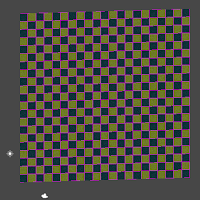For example.
Now I also promised collision meshes in this part. Collision meshes are really exactly the same as the meshes we made so far just without the textures. They also can't face the camera like the block textures do, they'll have to face up, left, right and down. We can't just go and add collision meshes to every block in the scene though because most of these are surrounded by other blocks, if there's no way to get to a block there's no need to spend time making it solid.

We'll start just making the colliders and think about how to implement them later. For testing you'll want to make your block array into a 1x1 array. Also make new variables colVertices and colTriangles, a new int colCount and a MeshCollider.
```

public List<Vector3> colVertices = new List<Vector3>();
public List<int> colTriangles = new List<int>();
private int colCount;

private MeshCollider col;
```

To use the MeshCollider you'll have to define it in Start() with:
```
col = GetComponent<MeshCollider> ();
```

And the UpdateMesh() function will need a few additions, firstly we make a temporary mesh to apply the collision mesh data to and then we apply it to the collision mesh. Then like with the other lists we need to clear the collision lists and reset the counter.
```

Mesh newMesh = new Mesh();
newMesh.vertices = colVertices.ToArray();
newMesh.triangles = colTriangles.ToArray();
col.sharedMesh= newMesh;

colVertices.Clear();
colTriangles.Clear();
colCount=0;
```

On to actually making the mesh, we're going to let collider generation happen in it's own function that we'll call for each block when we update the mesh so make a function called GenCollider with the parameters (int x, int y):

```void GenCollider(int x, int y){

}
```

We're now going to make squares just like before except that these will face up, left, right and down to make the squares we've drawn already be solid. You can probably guess what the code is going to look like. We'll be using colVertices and colTriangles instead of newVertices and newTriangles and we won't by using UVs because a collision model doesn't need a texture but otherwise these squares are made in the same way as our textures square.

```//Top
colVertices.Add( new Vector3 (x  , y  , 1));
colVertices.Add( new Vector3 (x + 1 , y  , 1));
colVertices.Add( new Vector3 (x + 1 , y  , 0 ));
colVertices.Add( new Vector3 (x  , y  , 0 ));

colCount++;

```

And call the GenCollider function for every block by putting it in the BuildMesh function in the for loops along with an if to check if the block is air:
``` void BuildMesh(){
for(int px=0;px<blocks.GetLength(0);px++){
for(int py=0;py<blocks.GetLength(1);py++){

//If the block is not air
if(blocks[px,py]!=0){

// GenCollider here, this will apply it
// to every block other than air
GenCollider(px,py);

if(blocks[px,py]==1){
GenSquare(px,py,tStone);
} else if(blocks[px,py]==2){
GenSquare(px,py,tGrass);
}
}//End air block check
}
}
}
```Your scene view should show this when run now. One face with an upward facing collider behind it.
Edit: Taryndactyl pointed out that displaying mesh colliders may be turned off by default in the editor, if you don't see a collider like above check Mesh Colliders in the gizmos menu:

So that code was just defining the points of the square and then creating the triangle data. The colCount is the same as the squareCount was to the last mesh code we did. Now I'll lay out the code for the other sides, there's really not much to learn in what numbers the vertices should be using; for me at least it's mostly a lot of trial and error, scribbling on paper and trying to visualize the four points' coordinates to figure out where each mesh should have its vertices. As long as you understand that each colVertices is a coordinate in 3d space that a corner of the cube uses and that the side that's facing you when you put down the triangle coordinates clockwise will be the solid one you've got it.

Before we add the other sides though let's move the triangle code to it's own small function because we're going to be using it so much, call it ColliderTriangles:
``` void ColliderTriangles(){
}
```

Good, now we can call that function instead of writing it out four times. Now all the sides for the collider should look like this:
```void GenCollider(int x, int y){

//Top
colVertices.Add( new Vector3 (x  , y  , 1));
colVertices.Add( new Vector3 (x + 1 , y  , 1));
colVertices.Add( new Vector3 (x + 1 , y  , 0 ));
colVertices.Add( new Vector3 (x  , y  , 0 ));

ColliderTriangles();

colCount++;

//bot
colVertices.Add( new Vector3 (x  , y -1 , 0));
colVertices.Add( new Vector3 (x + 1 , y -1 , 0));
colVertices.Add( new Vector3 (x + 1 , y -1 , 1 ));
colVertices.Add( new Vector3 (x  , y -1 , 1 ));

ColliderTriangles();
colCount++;

//left
colVertices.Add( new Vector3 (x  , y -1 , 1));
colVertices.Add( new Vector3 (x  , y  , 1));
colVertices.Add( new Vector3 (x  , y  , 0 ));
colVertices.Add( new Vector3 (x  , y -1 , 0 ));

ColliderTriangles();

colCount++;

//right
colVertices.Add( new Vector3 (x +1 , y  , 1));
colVertices.Add( new Vector3 (x +1 , y -1 , 1));
colVertices.Add( new Vector3 (x +1 , y -1 , 0 ));
colVertices.Add( new Vector3 (x +1 , y  , 0 ));

ColliderTriangles();

colCount++;

}
```You should see this; all four colliders.
Now, we have a working square with colliders. if you were to extend the size of the array for more squares however, you would run into an efficiency problem because every solid square is creating eight triangles. That's a lot more than we need so we need a way to only make these colliders when they face an empty block. For that we'll need a function to check the contents of a block.
``` byte Block (int x, int y){

if(x==-1 || x==blocks.GetLength(0) ||   y==-1 || y==blocks.GetLength(1)){
return (byte)1;
}

return blocks[x,y];
}
```
This is a simple function that checks if the block you're checking is within the array's boundaries, if not it returns 1 (Solid rock) otherwise it returns the block's value. This means that we can use this places where we're not sure that the block we're checking is within the level size. We'll use this in the collider function.

This is done by surrounding every collider side generation with an if that checks in the direction of the collider. ie. the left collider is only generated if the block to this block's left is air. Do it like this:
``` void GenCollider(int x, int y){

//Top
if(Block(x,y+1)==0){
colVertices.Add( new Vector3 (x  , y  , 1));
colVertices.Add( new Vector3 (x + 1 , y  , 1));
colVertices.Add( new Vector3 (x + 1 , y  , 0 ));
colVertices.Add( new Vector3 (x  , y  , 0 ));

ColliderTriangles();

colCount++;
}

//bot
if(Block(x,y-1)==0){
colVertices.Add( new Vector3 (x  , y -1 , 0));
colVertices.Add( new Vector3 (x + 1 , y -1 , 0));
colVertices.Add( new Vector3 (x + 1 , y -1 , 1 ));
colVertices.Add( new Vector3 (x  , y -1 , 1 ));

ColliderTriangles();
colCount++;
}

//left
if(Block(x-1,y)==0){
colVertices.Add( new Vector3 (x  , y -1 , 1));
colVertices.Add( new Vector3 (x  , y  , 1));
colVertices.Add( new Vector3 (x  , y  , 0 ));
colVertices.Add( new Vector3 (x  , y -1 , 0 ));

ColliderTriangles();

colCount++;
}

//right
if(Block(x+1,y)==0){
colVertices.Add( new Vector3 (x +1 , y  , 1));
colVertices.Add( new Vector3 (x +1 , y -1 , 1));
colVertices.Add( new Vector3 (x +1 , y -1 , 0 ));
colVertices.Add( new Vector3 (x +1 , y  , 0 ));

ColliderTriangles();

colCount++;
}

}
```

Now when you run it you should get a much more efficient collision mesh, go ahead and switch the array size back to 10 by 10.Colliders only along the top where the blocks are exposed to air.
With changes to the GenTerrain function you can see the side colliders in action too:Hmm... these are almost starting to look like cubes
If you want you can do the same to the block visuals as with the colliders and not render (Or render a different texture for) blocks that aren't exposed to air in order to hide ores and things. I'll let you figure that out.

That concludes part two, as always if there are any problems please leave a comment as soon as possible and I'll fix it. I'm open for any feedback. Feel free to follow me on twitter (@STV_Alex) or G+ to get updated when I post part three.

Here is a complete version of the code as of the end of part 2: Part 2 finished code

Next time we'll be using perlin noise to make more interesting terrain, caves and ores and adding functions to build/destroy blocks based on mouse clicks or collisions with the blocks (Like when a character hits a block with a hammer for example).

Edit: Thanks again to Taryndactyl on the unity forums and thanks to Wolli in the comments for pointing out some errors! Taryndactyl's post: Link

Part 3

1.Thanks for these! Really well written and helpful. Need part 3 though please :D

2.Thanks for these great tutorials.

Two error to report so far.

In part 1 we write:
newVertices.Add( new Vector3 (x , y , z ));
newVertices.Add( new Vector3 (x + 1 , y , z ));
newVertices.Add( new Vector3 (x + 1 , y-1 , z ));
newVertices.Add( new Vector3 (x , y-1 , z ));

However, you later the Zs to 0s. But not during tutorial 2, so there is a heap of errors when we first run the game in part 2 (before the collision section.)

Here's the second. In part 2 we add these lines:
newUV.Add(new Vector2 (tUnit * tStone.x, tUnit * tStone.y + tUnit));
newUV.Add(new Vector2 (tUnit * tStone.x + tUnit, tUnit * tStone.y + tUnit));
newUV.Add(new Vector2 (tUnit * tStone.x + tUnit, tUnit * tStone.y));
newUV.Add(new Vector2 (tUnit * tStone.x, tUnit * tStone.y)

But in your paste the last line is missing a semi-colon. I'm fortunately not copying and pasting (because I think that invalidates the point of a tutorial, I think.) I just managed to spot it.

Do you prefer "error reports" being posted here? Or is there somewhere better?

1.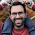Thanks Wolli, I've fixed what you pointed out. It's very helpful to have people who can manage problems like this and then let me know so I can make it easier for others. I think it's best to have these kinds of error reports in the comments, I fix the problems as soon as I can but someone else might stumble across the same thing before then and it's nice for them to be able to find a solution.

2.Dude this is awesome, step by step. . . Thank you so much.

3.This guide has been very helpful, i'm new to Unity and game programming in general and have been able to keep up so far with your guide.

4.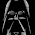Awsome, im learning heaps thanks.

5.This comment has been removed by the author.

1.Almost at the top of the page you print this:

public List<vector3> newVertices = new List<vector3>();
public List<int> newTriangles = new List<int>();
public List<vector2> newUV = new List<vector2>();

Having lower case Vector giving an error: "The type or namespace name `vector2' could not be found. Are you missing a using directive or an assembly reference?"

Switched it over to Capital V and the error was fixed. However, the next problem follows:

NullReferenceException
UnityEngine.Mesh.Clear ()

Meaning the Mesh is null, right?

This was solved by Wolli pointing it out: (In part 2 we add these lines)

newUV.Add(new Vector2 (tUnit * tStone.x, tUnit * tStone.y + tUnit));
newUV.Add(new Vector2 (tUnit * tStone.x + tUnit, tUnit * tStone.y + tUnit));
newUV.Add(new Vector2 (tUnit * tStone.x + tUnit, tUnit * tStone.y));
newUV.Add(new Vector2 (tUnit * tStone.x, tUnit * tStone.y)

Which is missing a " ); " at the end of the last line. (From his copy-paste atleast)

But I still have something wierd going on. The block on the top right corner is not generated for me. This I just cannot wrap my head around.. Any help?

6.I am at the part where I should first see the intial grid picture but I can not see it... If i put the original Start code back in it shows the single square. What should all of start look like by that point?

1.like you, I added the new UpdateMesh() method in the Update() method. Once I removed UpdateMesh(); from Update() I got the grid to display

7.On the list declarations you have:

public List newVertices = new List();

public List newUV = new List();

The v in vector should be V or it cannot refer to the struct and you should get an error.

8.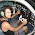wouldn't make more sense than when you put this:
byte Block (int x, int y){

if(x==-1 || x==blocks.GetLength(0) || y==-1 || y==blocks.GetLength(1)){
return (byte)1; // SHOULD RETURN 0 INSTEAD OF 1
}

return blocks[x,y];
}

What the comment says haha. because on the borders of the array there should be a collider. right?

1.You can't collide with something that you can't even get on, it's a check to see if it's out of boundaries.

2.It's a mistake on the tutorial. First he is assuming that it will return 1 but then he is using 0. Anyway I just tried with a bool insteaf of a byte:

bool Block (int x, int y)
{
if (x == -1 || x == bloques.GetLength (0) || y == -1 || y == bloques.GetLength (1))
{
return true;
}
return false;
}

For me is easier and don't conflict with this:

void GenTerrain()
{
bloks = new byte[10, 10];
int num_random;
for (int px = 0; px < bloques.GetLongLength(0); px++)
{
num_random = Random.Range(7,9);
for (int py=0; py < bloques.GetLongLength(1); py++)
{
switch (py)
{
case 9:
bloks[px,py]=0;
break;
case 8:
bloks[px,py]=1;
break;
default:
if (py == num_random)
{
bloks[px,py]=1;
}
else
{
bloks[px,py]=2;
}
break;
}
}
}
}

//Yes the code is modified a bit to my taste

9.Hi,

Thank you so much for taking the time to write this, you've helped me considerably. I haven't finished working my way through it all yet (After adding the collider, my mesh can no longer be seen)

I have picked up a couple of minor issues while following through...

Your GenSquare() method is assuming a "float z = 0;" - perhaps you copied it from a later version with a z param?

Also, your "List" should be "List" (casing issue)

I personally find the following a more readable way of specifying the uv - by factoring out your scale factor...

newUV.Add(tUnit * new Vector2(texture.x, 1 * texture.y + 1));
newUV.Add(tUnit * new Vector2(texture.x + 1, texture.y + 1));
newUV.Add(tUnit * new Vector2(texture.x + 1, texture.y));
newUV.Add(tUnit * new Vector2(texture.x, 1 * texture.y));

Of course that's purely a matter of style.

10.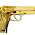I'm having trouble, where we add the public byte thing, I get lost after that bit.

11.The following error occurred:
NullReferenceException
PolygonGenerator.UpdateMesh () (at Assets/Scripts/PolygonGenerator.cs:64)
PolygonGenerator.Start () (at Assets/Scripts/PolygonGenerator.cs:37)
apparently does not recognize my unity MeshColider ...

12.ok so for those who encounter the NullReferenceException, check the error line, it means the objects you're refering to doesn't exists. I had it because I added the line :

col = GetComponent ();

after the 3 other functions called in the Start()

so trying to assign newMesh to col.sharedMesh couldn't work as col didn't existed yet.

hope it helps.

13.Hey man, tks so much, your tutorials is great.

14.I'm not sure what I'm missing here but only the top collision meshes seem to be rendering. Thinking it was Unity simply wasn't rendering the gizmo for the colliders, I tested it with the built-in character controller. My character can stand on top of the blocks but goes through them from all sides. I've reviewed the code a few times but since I don't want to copy/paste, I don't know what I'm missing.

Also, I'm not sure what is supposed to be controlling the array size for how many blocks it's rendering at once. I don't recall it being pointed out in your code.

Any help would be appreciated. Thanks in advance.

1.Doing some further sleuthing, I copy and pasted the sample code and got results that more closely matched the last image in the tutorial. However, while I do seem to get the collision meshes on the insides (between the missing column 5), the front collision meshes are still missing. Is there perhaps some setting I need to change on the Mesh Collider Component of my Game Object? I looked back at the first tutorial and my settings seemed to match verbatim.

2.This is several years too late, but maybe it will help someone in 2017+.

The reason why the GenColliders is producing colliders in between the two different sets of blocks in the example image, is that the block in between is literally an "air" block.

If you go outside of the byte[x,y] limits, then it's empty space, and won't render colliders. This is why the top of the terrain object has colliders, but the left, right, and bottom edges do not: There are air blocks above the ground, but not outside the array, or below it.

Another way of saying it: Colliders only appear if there's a block edge INSIDE the array. Anything outside the limits of the array will not produce a collider.

15.I have a question. Are these supposed to be individual scripts or one long script? You never explained it and the number scale on the left insinuates they're separated.

1.For now I think that all is in the same scripts. The number on the left are from the code viewer use in the blog. So this is not the real number of his own script. I think that if you really want to have a big terrain generation you should split the code in multiple scripts. I think i'll will do it for my project. Like a script to generate the mesh, one to apply texture on it, and another one to create the collision meshes. Hope this answer will help you :)

16.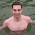This is the best dynamic mesh gen tutorial ever!!! Thanx! =)

17.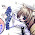someone can help me to fix it i have see this tutorial but i dont know what is this error

Mesh.uv is out of bounds. The supplied array needs to be the same size as the Mesh.vertices array.
UnityEngine.Mesh:set_uv(Vector2[])
NewGame:UpdateMesh() (at Assets/NewGame.cs:63)
NewGame:Start() (at Assets/NewGame.cs:54)

18.I keep getting this error:

Failed setting triangles. Some indices are referencing out of bounds vertices.
UnityEngine.Mesh:set_triangles(Int32[])
terrain_PolygonGenerator:updateMesh() (at Assets/Standard Assets/Custom Scripts/Voxel based scripts/terrain_PolygonGenerator.cs:232)
terrain_PolygonGenerator:Start() (at Assets/Standard Assets/Custom Scripts/Voxel based scripts/terrain_PolygonGenerator.cs:52)

1.This comment has been removed by the author.

2.It's because on GenSquare(int x, int y, Vector2 texture) you are creating less or more triangles than needed. Post here your code and I may search the problem

19.Hey man thanks for the tut I think that it would be easier for beginners to understand if you generated the vertices for the collider squares in the same order top -> top right -> bottom right -> bottom left for each one just because it wouldn't be a question to wonder about. Thanks again!

20.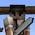This comment has been removed by the author.

21.Hi,
I have been following the tutorial, but when I get to the part where a collider is supposed to be visible in game mode, it does not appear. I checked MeshCollider in gizmos. If anyone finds my error, please let me know. I have been stuck for two days now.

using System.Collections;
using System.Collections.Generic;

public class PolygonGenerator : MonoBehaviour {

private int squareCount = 0;
private float tUnit = .25f;
private Vector2 tStone = new Vector2 (0, 0);
private Vector2 tGrass = new Vector2 (0, 1);

public byte[,] blocks;
//contains every vertex that will be rendered
public List newVertices = new List();
//Triangles tell Unity how to build each section of the mesh
//joining the vertices
public List newTriangles = new List ();
// UV list tells unity how the texture is alligned on each polygon
List newUV = new List();
// A mesh is made out of the above components
private Mesh mesh;

public List colVertices = new List();
public List colTriangles = new List();
private int colCount;

private MeshCollider col;

// Use this for initialization
void Start () {

mesh = GetComponent ().mesh;
col = GetComponent ();

GenTerrain ();
BuildMesh ();
UpdateMesh ();

}

// Update is called once per frame
void Update () {

}

void UpdateMesh (){
mesh.Clear ();
mesh.vertices = newVertices.ToArray ();
mesh.triangles = newTriangles.ToArray ();
mesh.uv = newUV.ToArray ();
mesh.Optimize ();
mesh.RecalculateNormals ();
squareCount = 0;

Mesh newMesh = new Mesh();
newMesh.vertices = colVertices.ToArray();
newMesh.triangles = colTriangles.ToArray();
col.sharedMesh= newMesh;

colVertices.Clear();
colTriangles.Clear();
colCount=0;
}

void GenSquare(int x, int y, Vector2 texture){
newVertices.Add (new Vector3 (x, y, 0));
newVertices.Add (new Vector3 (x + 1, y, 0));
newVertices.Add (new Vector3 (x + 1, y - 1, 0));
newVertices.Add (new Vector3 (x, y - 1, 0));

newTriangles.Add ((squareCount * 4) + 1);
newTriangles.Add ((squareCount * 4) + 3);
newTriangles.Add ((squareCount * 4) + 1);
newTriangles.Add ((squareCount * 4) + 2);
newTriangles.Add ((squareCount * 4) + 3);

newUV.Add (new Vector2 (tUnit * texture.x, tUnit * texture.y + tUnit));
newUV.Add (new Vector2 (tUnit * texture.x + tUnit, tUnit * texture.y + tUnit));
newUV.Add (new Vector2 (tUnit * texture.x + tUnit, tUnit * texture.y));
newUV.Add (new Vector2 (tUnit * texture.x, tUnit * texture.y));
squareCount++;
}

void GenTerrain(){
blocks = new byte[1, 1];

for (int px = 0; px < blocks.GetLength(0); px++) {
for (int py = 0; py < blocks.GetLength(1); py++){

if ( py == 0){
blocks[px, py] = 2;
}
else if (false){
blocks[px, py] = 1;
}
}
}
}

void BuildMesh(){
for (int px=0; px<blocks.GetLength(0); px++) {
for (int py=0; py<blocks.GetLength(1); py++) {

//If the block is not air
if (blocks [px, py] != 0) {

// GenCollider here, this will apply it
// to every block other than air
GenCollider (px, py);

if (blocks [px, py] == 1) {
GenSquare (px, py, tStone);
} else if (blocks [px, py] == 2) {
GenSquare (px, py, tGrass);
}
}//End air block check
}
}
}
void GenCollider(int x, int y){
colVertices.Add( new Vector3 (x , y , 1));
colVertices.Add( new Vector3 (x + 1 , y , 1));
colVertices.Add( new Vector3 (x + 1 , y , 0 ));
colVertices.Add( new Vector3 (x , y , 0 ));

colCount++;
}
}

1.This comment has been removed by the author.

2.I'm having the same problem.
If I run the game, I can see the textured blocks (in game view).
But on scene view nothing shows up.
I've checked gizmos, settings, selections, etc...
Could it be a problem of Unity 4.5?

3.Ok, stupid thing, solved it.

As i'm using a small display, my scene view and game view share the same frame (can't se both at the same time), like by default.

OF COURSE nothing will show up in scene view while i'm not in "game mode". If anyone had the same retarded problem :P just split the frame, make sure you can view both SCENE and GAME. Then you'll see your mesh colliders.

;D

22.I'm having trouble with the part before all the collision stuff. I cannot get the code to render more than 10x6 squares. His initial example image shows 10x6 squares with a byte array of [10,10] (which is odd to me), but then he says we can expand it to generate a larger grid.

I've tried changing the byte array in GenTerrain() like so:

void GenTerrain() {
blocks = byte[50,50];

// for loops like in example
}

This still gives a 10x6 grid, instead of 50x50 grid. What's wrong?

1.Nevermind, I just figured it out. The for loop stops at py==5. Changing it to py>=5 fixes it.

23.Not sure if I've setup Unity correctly, but when I run this code, why does the first row of tiles start at y==-1?

ie, the first tile has vertices at (0,0) (1,0) (1,-1)(0,-1).

How can we change this to have the tiles begin generating at y==0?

24.This is an absolutely brilliant tutorial! Well done explaining difficult concepts in such a simple and easy-to-follow way. I'm not following step-by-step, but looking at how you do things to help me implement my own system.

Thanks so much! Brilliant work!

25.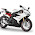This comment has been removed by the author.

26.I think there is a mistake in the function to check contents of a block. It should return 0 instead of 1? Otherwise I don't get any colliders showing at all. I could be wrong but here is the code I corrected:

byte Block (int x, int y){

if(x==-1 || x==blocks.GetLength(0) || y==-1 || y==blocks.GetLength(1)){
return (byte)0;
}

return blocks[x,y];
}

27.Hello, this is an awesome tutorial, but just wondering if it is possible to make the curvy shape of perlin sound apply to all 4 corners of the rectangle to make it look more like a spheroid?
I got this crazy idea but procedural generation is not my strongest part as I just started learning.

28.This comment has been removed by the author.